# Sample Data: Redwood Saplings

Locations of redwood saplings (young slender trees), annotated with binary indicator

## Details

Locations of redwood saplings (young slender trees) in the observation region Rectangle[{0, 0}, {1, 1}], annotated with binary categorical indicator (0 or 1).

## Examples

### Basic Examples (1)

 In:=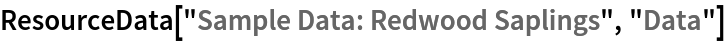Out=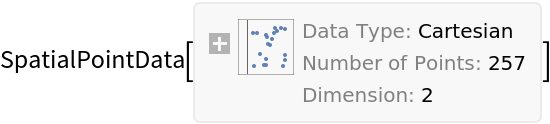Retrieve the default content:

 In:=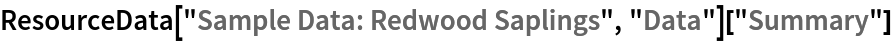Out=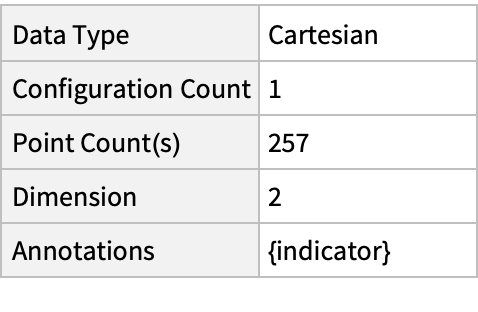### Visualizations (3)

Plot the spatial point data:

 In:=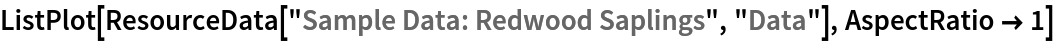Out=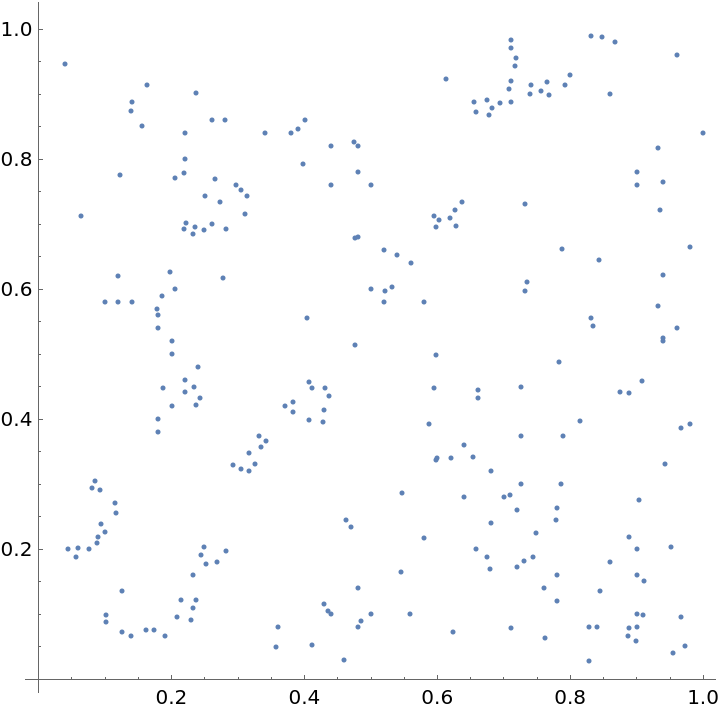Visualize the data points with annotations:

 In:=Out=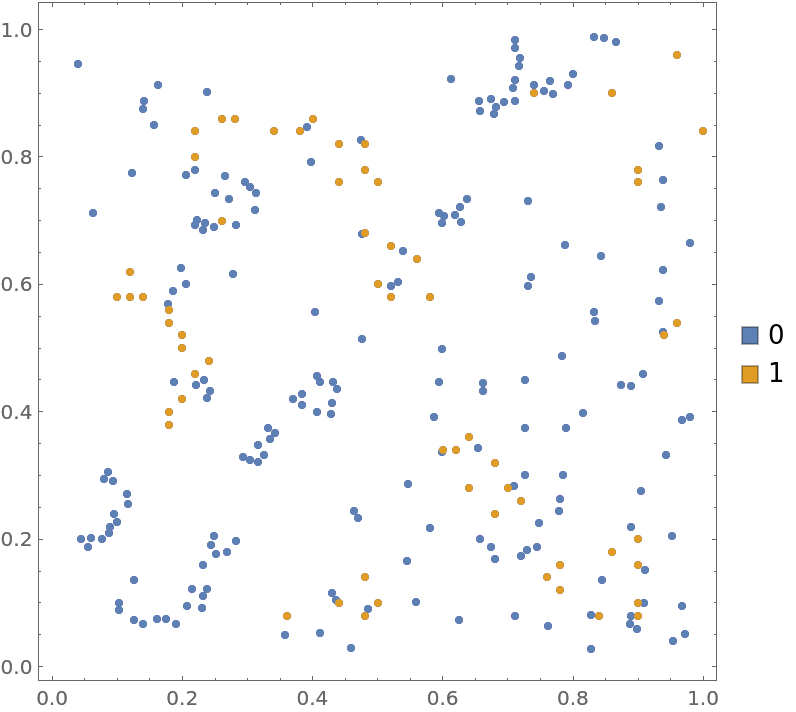Visualize the smooth point density of the data:

 In:=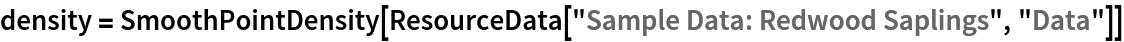Out=In:=Out=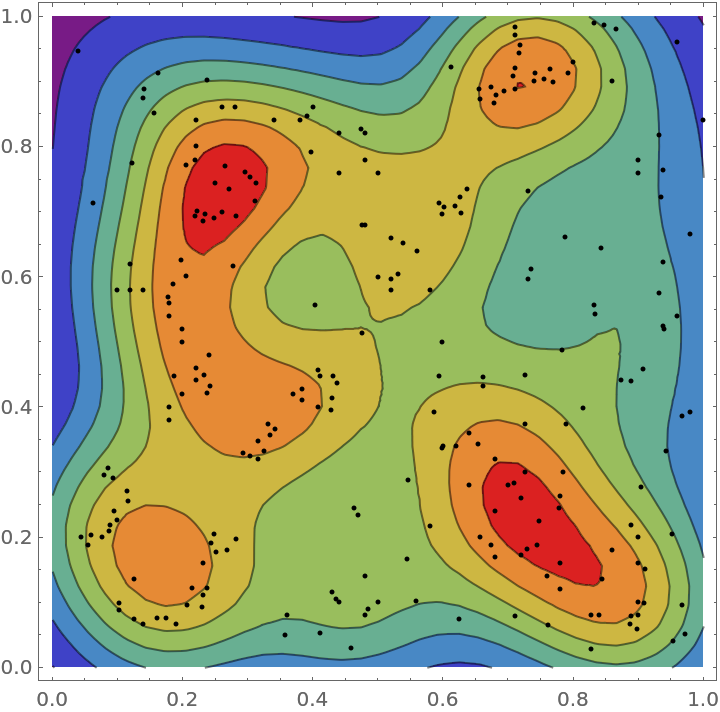### Analysis (4)

Compute probability of finding a point within given radius of an existing point - NearestNeighborG is the CDF of the nearest neighbor distribution:

 In:=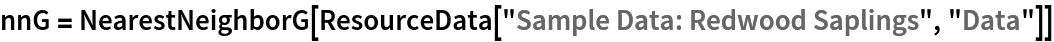Out=In:=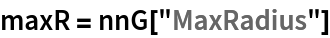Out=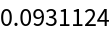In:=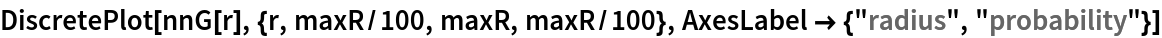Out=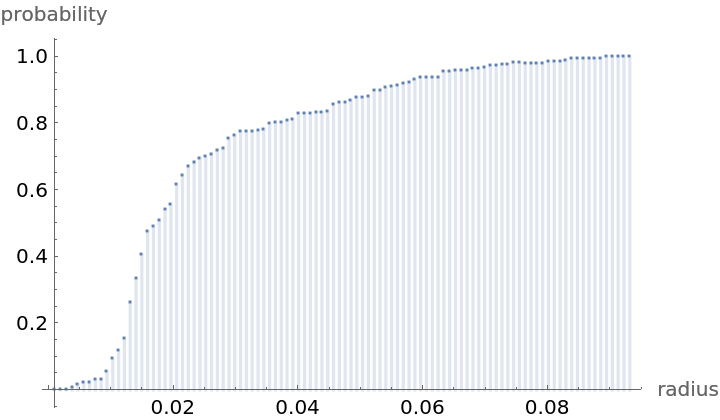NearestNeighborG as the CDF of nearest neighbor distribution can be used to compute the mean distance between a typical point and its nearest neighbor - the mean of a positive support distribution can be approximated via a Riemann sum of 1- CDF. To use Riemann approximation create the partition of the support interval from 0 to maxR into 100 parts and compute the value of the NearestNeighborG at the middle of each subinterval:

 In:=Now compute the Riemann sum to find the mean distance between a typical point and its nearest neighbor:

 In:=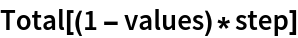Out=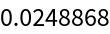Test for complete spatial randomness:

 In:=Out=Gosia Konwerska, "Sample Data: Redwood Saplings" from the Wolfram Data Repository (2022)